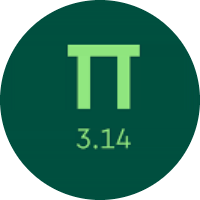# Knowing the Fractions and Their Operations

In this quiz, you will get to know about the basics of fractions. You will find problems of equivalent fractions, writing fractions in the lowest terms, improper, mixed fractions, comparing fractions, and putting fractions in order. You will also find different types of problems on additions and subtractions of fractions.

Start Quiz

Type the missing number that makes these fractions equal: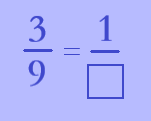1

3

6

What is the least common denominator of 1/12 and 1/6?

6

9

12

Which fraction is greater?

1/11

2/11

3/11

Write 19/50 as a decimal number.

0.38

0.19

0.95

Write 0.35 as a fraction.

7/10

7/20

7/15

Put these numbers in order from least to greatest.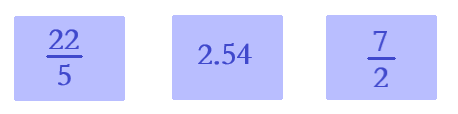7/2<22/5<2.54

2.54<7/2<22/5

2.54<22/5<7/2

Evaluate.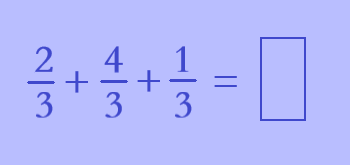5/3

4/3

7/3

Evaluate.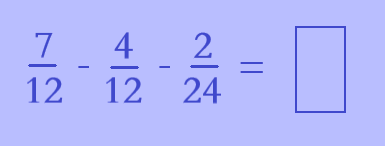1/6

2/6

3/12

Which sign makes the statement true?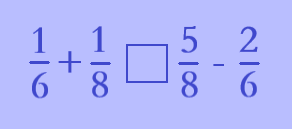>

=

<

Evaluate.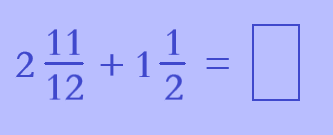51/12

53/12

47/12

Quiz/Test Summary
Title: Knowing the Fractions and Their Operations
Questions: 10
Contributed by: